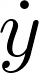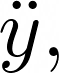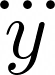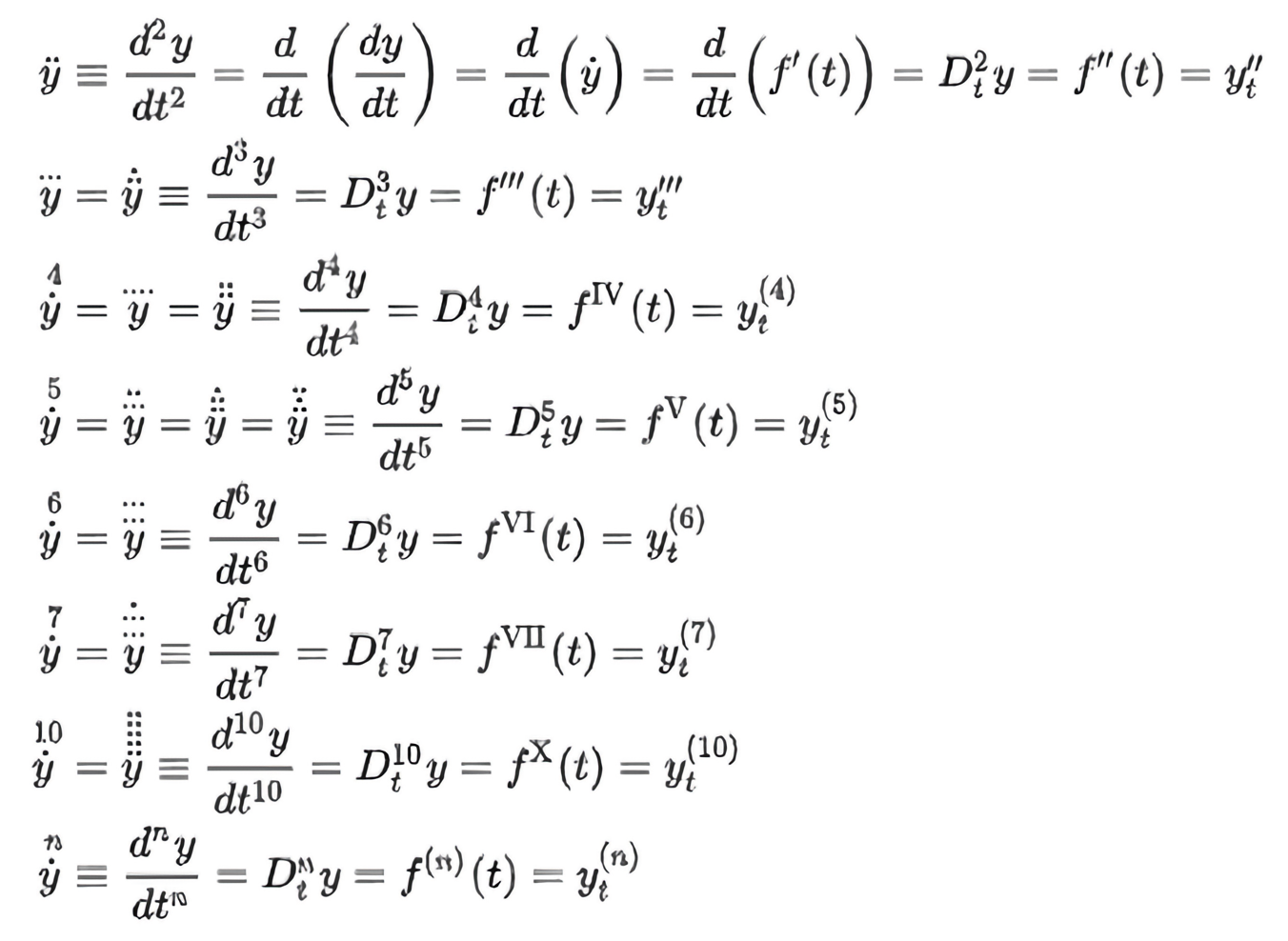## NewtonSir Isaac Newton PRS (25 December 1642 – 20 March 1726) was an English mathematician, physicist, astronomer, theologian, and author who is widely recognised as one of the most influential scientists of all time and as a key figure in the scientific revolution. His book Philosophiæ Naturalis Principia Mathematica , first published in 1687, laid the foundations of classical mechanics. Newton also made seminal contributions to optics, and shares credit with Gottfried Wilhelm Leibniz for developing the infinitesimal calculus.

Newton’s work has been said “to distinctly advance every branch of mathematics then studied.” His work on the subject usually referred to as fluxions or calculus, seen in a manuscript of October 1666, is now published among Newton’s mathematical papers.

Newton’s notation for differentiation (also called the dot notation, or sometimes, rudely, the flyspeck notation for differentiation) places a dot over the dependent variable. That is, if y is a function of t, then the derivative of y with respect to t isHigher derivatives are represented using multiple dots, as in:Newton extended this idea quite far: